Next: Spacecraft Elements Up: Planetocentric Systems Previous: Planetocentric Systems

### Jovian Systems

Since we have used different Jovian coordinate systems in previous work (Krupp et al., 1993) we include a description of these systems. Most of these systems are discussed in Dessler (1983). The Jovian pole of rotation is defined by the values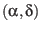given for "Jupiter III" in Table.6. The transformation from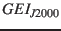can be calculated from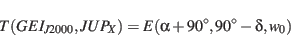(38)

where the prime meridian angle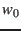is given in the following table. Note that longitudes are counted left-handed (clockwise) from the prime meridian in the following Jovian systems.

• System I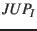, mean atmospheric equatorial rotation [S. Table 15.7]
+Z-axis: Pole of rotation. p-angle: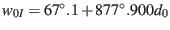• System II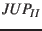, mean atmospheric polar rotation [S. Table 15.7]
+Z-axis: Pole of rotation. p-angle: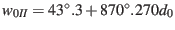• System III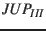, magnetospheric rotation [S. Table 15.7]
 +Z-axis: Pole of rotation. p-angle: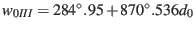Transform: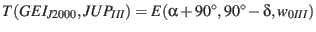This is the 1965 definition of System III, the Pioneer missions used the 1957 definition: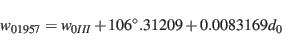which can be calculated from eqn.7c in Seidelmann and Divine (1977) and was originally defined by the magnetospheric rotation period.
• System III fix Sun Line
+Z-axis: Pole of rotation. +Y-axis: cross-product of +Z-axis and vector (Jupiter-Sun)
• Magnetic Dipole System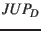(Dessler, 1983)
+Z-axis:    dipole axis defined by its System III latitude and longitude: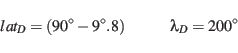+X-axis: intersection of System III prime meridian and magnetic equator. Transform: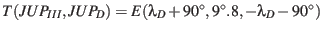(approximately).
• Centrifugal System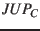(Dessler, 1983)
 +Z-axis: centrifugal axis defined by its System III latitude and longitude: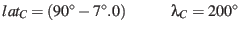+X-axis: intersection of System III prime meridian and centrifugal equator. Transform: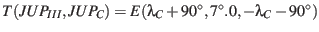(approximately).
• Magnetic Dipole System fix Sun line
+Z-axis: dipole axis. +Y-axis: cross-product of +Z-axis and vector (Jupiter-Sun)
• Magnetic Dipole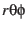System
+X-axis: vector (Jupiter-S/C) +Z-axis: (dipole axis)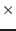+X-axis
This system depends on the S/C-position.Next: Spacecraft Elements Up: Planetocentric Systems Previous: Planetocentric Systems
Markus Fraenz 2017-03-13